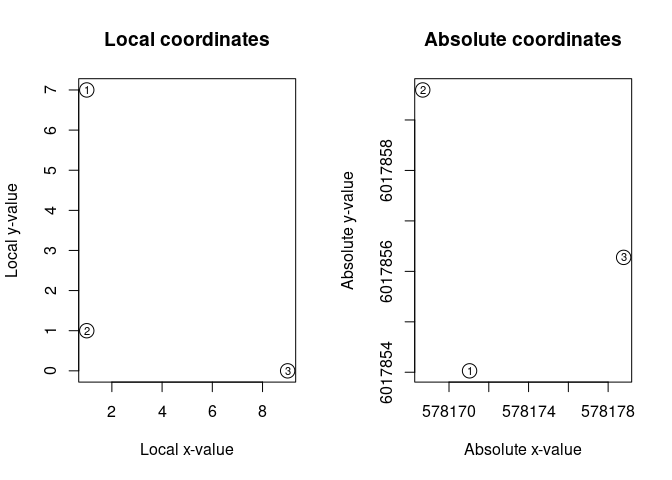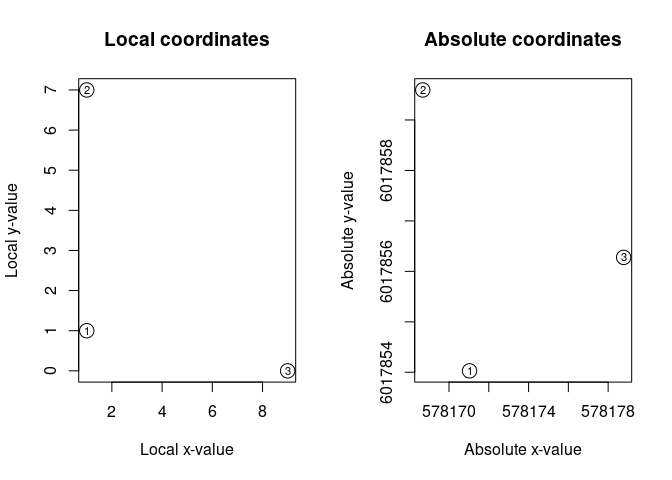# recexcavAAR: Vignette >>Transforming coordinates<<

#### January 2017

This vignette gives an overview on the topic of 2D-Coordinate Transforming with the now in recexcevARR implemented cootrans-function.

## Background

In archaeology you often have to deal with data acquired in an era before DGPS and tacheometry became the common surveying method. Even today some excavations use the traditional methods. These data are mostly recorded in local defined grids which are often not suitable for mapping in Geographic Information Systems. The common method to solve that problem is moving and rotating the survey points by hand until they fit the reality.
The cootrans-function provides a method to transform a set of local acquired coordinates into projected ones under given circumstances using mathematical calculations.

## Requirements

This transformation method works only if you have at least two pairs of coordinates of which you know the local and the projected coordinates. Note that these coordinates have to be projected not geographic1.

## Limitations

• This method provides a two-dimensional transformation so only X- and Y-values will be calculated (4-Parameter-Helmert-Transformation).
• This method is not suitable for datum transformations (e.g. converting from UTM to national grids).

## Example: A simple transformation

library(recexcavAAR)

For this example we use a simple dataset. At first we have the data of the initial surveying with the three major measuring points placed by the surveyor. These are our projected coordinates (in this example UTM-coordinates).

proj <- data.frame(
E = c(578171.033, 578168.680, 578178.780),
N = c(6017854.028, 6017859.595, 6017856.277)
)

After we constructed our own local grid, we add the corresponding local coordinates to the projected ones.

coord <- data.frame(
proj,
X = c(1, 1, 9),
Y = c(7, 1, 0)
)

This is the data table we need to successfully perform a transformation for all local coordinates we acquire throughout the excavation.

Now we add some data e.g. the corners of our excavation trench or the artifacts we excavated.

measured_points <- data.frame(
x = c(1,6,1,6),
Y = c(3,3,5,5),
point = c("corner1", "corner2", "corner3", "corner4")
)

And now let’s use the cootrans-function to calculate the UTM-coordinates of our trench corners.

absolute_data <- cootrans(coord, c(3,4,1,2), measured_points, c(1,2))
## Transformation:
##  local centroid: 3.66666666666667 2.66666666666667
##  absolute centroid: 578172.831 6017856.63333333
##  scale: 1.08105445197455
##  rotation arc: 216.864428620413
## Warning in cootrans(coord, c(3, 4, 1, 2), measured_points, c(1, 2)): High
## deviations! Some coordinates may be mapped incorrectly.

The first parameter in this function is our data.frame for the transformation. The directional shifts, rotation arcs and the scale will be calculated from these information.
The second parameter is a vector of the column indices of our transformation table in the specific order:

1. the local x-value (east-value)

2. the local y-value (north-value)

3. the projected x-value (east-value)

4. the projected y-value (north-value)

The third parameter is the data.frame with our measured local coordinates which should be transformed.
Our last parameter is a vector of the column indices of our dataset in order:

1. the local x-value (east-value)

2. the local y-value (north-value)

The cootrans-function returns the original data.frame with two additional columns with the calculated projected coordinates and shows information about the transformation.

In this case we get an addtional warning message of high deviations within the calculation. The most likely mistake is the incorrect assignement of local and corresponding projected coordinates. This does not have to be the reason for the triggered warning message because the internal control mechanism is rather strict. But to check the case of incorrectly defined points we can use the additional parameter “checking” which is set FALSE by default.

check_data <- cootrans(coord, c(3,4,1,2), measured_points, c(1,2), checking = TRUE)
## Warning in cootrans(coord, c(3, 4, 1, 2), measured_points, c(1, 2),
## checking = TRUE): High deviations! Some coordinates may be mapped
## incorrectly.In this case the function will display a combined plot with the mapped local and projected coordinates. Every survey point is labled with an index number of their row.
Addtionally the function now returns our transformation table with the calculated scales and roation arcs (in degrees) for each pair of coordinates.

check_data
##          E       N X Y scalation  rotation
## 1 578171.0 6017854 1 7 0.6221418 246.21795
## 2 578168.7 6017860 1 1 1.6215558  67.51258
## 3 578178.8 6017856 9 0 0.9994657 336.86276

If we display our check_data, we will notice the scale of pair three seems fine. In both (local and absolute) grids, we use metres as units, so the scale schould be around 1. The scale of point one and two do not match this assumption, also the rotations arcs differ too much.
The displayed plots and the check_data show that the local points with the indices 1 and 2 are not corresponding with the projected ones. So they are likely mixed up. In this case we simply rebuild the transformation data.frame:

corr_coord <- data.frame(
proj,
X = c(1, 1, 9),
Y = c(1, 7, 0)
)

As shown we only interchanged the Y-value of the first and second coordinate. Now we run the cootrans-function for the second time and check our transformation:

check_data <- cootrans(corr_coord, c(3,4,1,2), measured_points, c(1,2), checking = TRUE)
## Transformation:
##  local centroid: 3.66666666666667 2.66666666666667
##  absolute centroid: 578172.831 6017856.63333333
##  scale: 1.00276297710336
##  rotation arc: 336.864428620413And everything seems fine. No warning message pops up and the check_data show all scales and rotaions arcs around the same values.
So now let’s use the cootrans-function one last time to get our absolute data for the excavation trench corners.

absolute_data <- cootrans(corr_coord, c(3,4,1,2), measured_points, c(1,2))
## Transformation:
##  local centroid: 3.66666666666667 2.66666666666667
##  absolute centroid: 578172.831 6017856.63333333
##  scale: 1.00276297710336
##  rotation arc: 336.864428620413

At this point we can proceed with further analyses of our now georeferenced spatial data.

Attention!
The warning message and checking parameter only work reasonable if there are more than two given coordinate pairs. In case of only two points and incorrect defined coordinates the algorithm will just turn all of the measured points by 180°. So always check your output!

1 For those who are not familiar with these terms: projected coordinates use a grid system such as UTM. Geographic coordinates are e.g. “lon-lat”-coordinates.